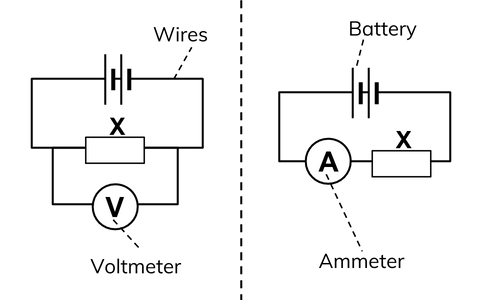Question

# A charge of 2C moves between two plates maintained at a potential difference of 1V. What is the energy acquired by the charge?

Open in App
Solution

## Potential difference: A potential difference is a difference that is the same as voltage.It is equal to the amount of current multiplied by the resistance.One volt of potential difference is equal to the one joule of energy.A diagram to represent the potential difference:Potential difference formula:$V=\frac{W}{Q}$Here, a potential difference is equal to $V=1v$Work done=Energy acquired=WAnd, the change is equal to $Q=2C$Now put the given value in the equation.$1V=\frac{W}{2C}$Now, $W=1V×2C$So, $W=2J$Therefore, the energy acquired by the change is $2Joule$Suggest Corrections0Similar questions